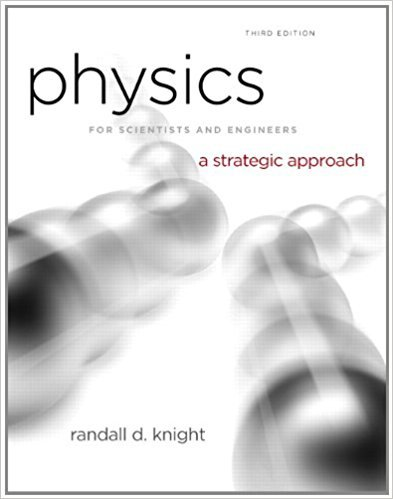×
Log in to StudySoup
Get Full Access to Physics For Scientists And Engineers: A Strategic Approach With Modern Physics - 3 Edition - Chapter 15 - Problem 73cp
Join StudySoup for FREE
Get Full Access to Physics For Scientists And Engineers: A Strategic Approach With Modern Physics - 3 Edition - Chapter 15 - Problem 73cp

Already have an account? Login here
×
Reset your password

# A cylinder of density ? o, length l, and cross-sectionISBN: 9780321740908 69

## Solution for problem 73CP Chapter 15

Physics for Scientists and Engineers: A Strategic Approach with Modern Physics | 3rd Edition

• Textbook Solutions
• 2901 Step-by-step solutions solved by professors and subject experts
• Get 24/7 help from StudySoup virtual teaching assistantsPhysics for Scientists and Engineers: A Strategic Approach with Modern Physics | 3rd Edition

4 5 1 238 Reviews
11
4
Problem 73CP

Problem 73CP

A cylinder of density ρ o, length l, and cross-section area A floats in a liquid of density ρ f with its axis perpendicular to the surface. Length h of the cylinder is submerged when the cylinder floats at rest.

a. Show that h = (ρ 0 /ρ f )l.

b. Suppose the cylinder is distance y above its equilibrium position. Find an expression for (F net)y, the y -component of the net force on the cylinder. Use what you know to cancel terms and write this expression as simply as possible.

c. You should recognize your result of part b as a version of Hooke’s law. What is the “spring constant” k ?

d. If you push a floating object down and release it, it bobs up and down. So it is like a spring in the sense that it oscillates if displaced from equilibrium. Use your “spring constant” and what you know about simple harmonic motion to show that the cylinder’s oscillation period ise. What is the oscillation period for a 100-m-tall iceberg (ρ ice = 917 kg/m3 ) in seawater?

Step-by-Step Solution:

Solution

Step 1 of 7

In this problem, we have to find an expression for, then we have to find an expression for net force, from the Hooke’s law we have to identify the spring constant, then show that the cylinder’s oscillation is period and finally find the oscillation period.

Step 2 of 7

Step 3 of 7

##### ISBN: 9780321740908

Unlock Textbook Solution

Enter your email below to unlock your verified solution to:

A cylinder of density ? o, length l, and cross-section